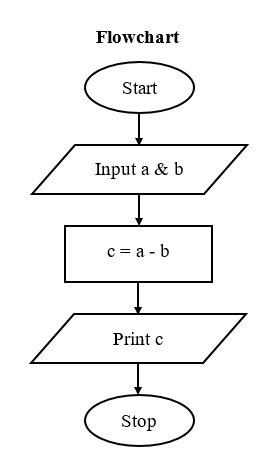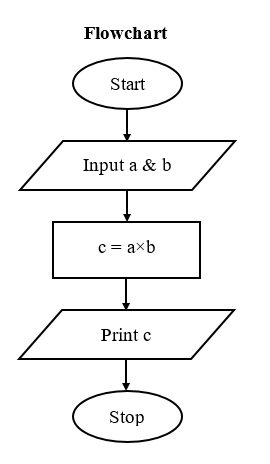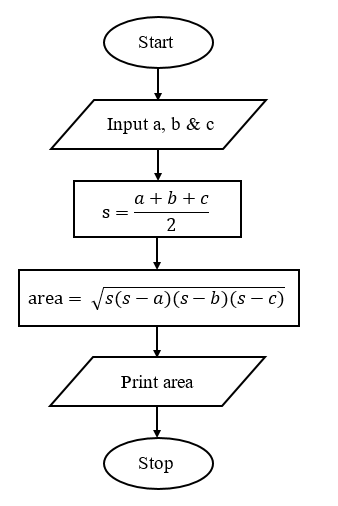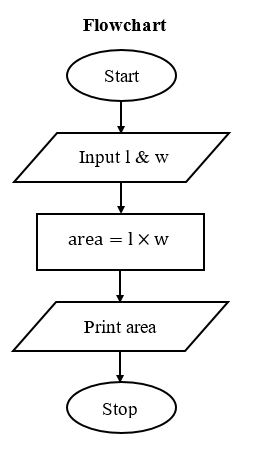Fifth Chapter Lesson-5 Basic Mathematical problem related algorithm & flowchart.

At the end of this lesson-

• 1. You will be able to write algorithm & flowchart for adding two numbers.
• 2. You will be able to write algorithm & flowchart for subtracting two numbers.
• 3. You will be able to write algorithm & flowchart for multiplying two numbers.
• 4. You will be able to write algorithm & flowchart for dividing two numbers.
• 5. You will be able to write algorithm & flowchart for converting temperature from Celsius to Fahrenheit.
• 6. You will be able to write algorithm & flowchart for converting temperature from Fahrenheit to Celsius.
• 7. You will be able to write algorithm & flowchart for determining area of a triangle where base and height have been given.
• 8. You will be able to write algorithm & flowchart for determining area of a triangle where three arms have been given.
• 9. You will be able to write algorithm & flowchart for determining area of a rectangle.
• 10. You will be able to write algorithm & flowchart for determining area of a circle.

1. Algorithm & flowchart for adding two numbers.

• Algorithm
• Step-1: Start
• Step-2: Input two numbers in variable a & b
• Step-3: Calculate c=a+b
• Step-4: Print value of c
• Step-5: StopPractice: Write an algorithm & flowchart for adding five integer numbers.

2. Algorithm & flowchart for subtracting two numbers.

• Algorithm
• Step-1: Start
• Step-2: Input two numbers in variable a & b
• Step-3: Calculate c=a-b
• Step-4: Print value of c
• Step-5: Stop3. Algorithm & flowchart for multiplying two numbers.

• Algorithm
• Step-1: Start
• Step-2: Input two numbers in variable a & b
• Step-3: Calculate c=a×b
• Step-4: Print value of c
• Step-5: StopPractice: Write an algorithm & flowchart for multiplying five integer numbers.

4. Algorithm & flowchart for dividing two numbers.

• Algorithm
• Step-1: Start
• Step-2: Input two numbers in variable a & b
• Step-3: Calculate c=a/b
• Step-4: Print value of c
• Step-5: StopPractice: Write an algorithm & flowchart for determining average of five integer numbers.

5. Algorithm & flowchart for converting temperature from Celsius to Fahrenheit.

Relation among different temperature scale C/5 = F-32 / 9 = K-273 / 5

• Algorithm
• Step-1: Start
• Step-2: Input Celsius temperature in variable C
• Step-3: Calculate F=(9C/5)+32
• Step-4: Print value of F
• Step-5: StopPractice: Write an Algorithm & flowchart for converting temperature from Celsius to Kelvin.

6. Algorithm & flowchart for converting temperature from Fahrenheit to Celsius.

Relation among different temperature scale C/5 = F-32 / 9 = K-273 / 5

• Algorithm
• Step-1: Start
• Step-2: Input Fahrenheit temperature in variable F
• Step-3: Calculate C=((F-32)5)/9
• Step-4: Print value of C
• Step-5: StopPractice: Write an Algorithm & flowchart for converting temperature from Fahrenheit to Kelvin.

7. Algorithm & flowchart for determining area of a triangle where base and height have been given.

Theory for determining the area of a triangle where base and height of triangle have been given,  Area=1/2×base×height

• Algorithm
• Step-1: Start
• Step-2: Input base and height of triangle in variable b & h
• Step-3: Calculate  area=1/2×b×h
• Step-4:  Print value of area
• Step-5: Stop8. Algorithm & flowchart for determining area of a triangle where three arms have been given.

Theory for determining the area of a triangle where three arms of triangle are a, b and c,                           Area= √(s(s-a)(s-b)(s-c))   [ s= half of perimeter]

half of perimeter, s=(a+b+c)/2

• Algorithm
• Step-1: Start
• Step-2: Input three arms of a triangle in variable a, b and c
• Step-3: Calculate s=(a+b+c)/2
• Step-4: Calculate area= √(s(s-a)(s-b)(s-c))
• Step-5: Print value of area
• Step-6: Stop

Flowchart:9. Algorithm & flowchart for determining area of a rectangle.

Theory for determining area of rectangle where length and width have been given, Area=length×width

• Algorithm
• Step-1: Start
• Step-2: Input length and width of rectangle in variable l and w
• Step-3: Calculate area=l×w
• Step-4: Print value of area
• Step-5: StopPractice: Write an Algorithm & flowchart for determining area of a parallelogram.

Practice: Write an Algorithm & flowchart for determining area of a square.

10. Algorithm & flowchart for determining area of a circle.

Theory for determining area of a circle where radius r, Area=πr2

• Algorithm
• Step-1: Start
• Step-2: Input radius of circle in variable r
• Step-3: Calculate area=πr2
• Step-4: Print area
• Step-5: StopLesson Evaluation-

Knowledge Based Questions:

Comprehension Based Questions:

Creative Questions:

Multiple Choice Questions:

Written by,